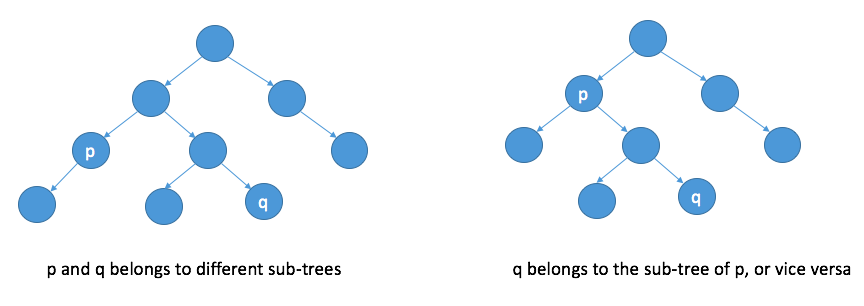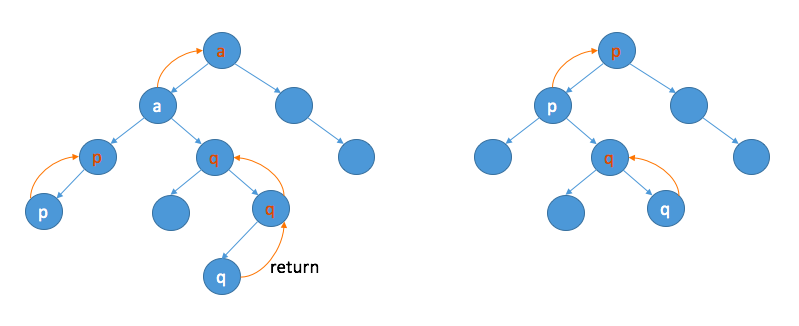A binary tree is a tree where each node has at most two children. Suppose the depth of a binary tree is k, then it has at most 2^k children.
A complete binary tree is a binary tree where every level (except the last) is completely filled, and all nodes at the last level are as far left as possible.
A full binary tree is a complete binary tree where every node other than the leaves has two children.

This post is about some classic problems relating to the binary tree. Most of the problems can be solved using DFS.

## I. Traversal

### i. Pre-order traversal

Leetcode: 144. Binary Tree Preorder Traversal.

Recursive solution:

Itervative solution:

### ii. In-order traversal

Leetcode: 94. Binary Tree Inorder Traversal.

Recursive solution:

Itervative solution:

Or use a set to record the nodes which have been processed (as shown in iii).

### iii. Post-order traversal

Leetcode: 145. Binary Tree Postorder Traversal.

Recursive solution:

Itervative solution:

### iv. Construct a binary tree using pre-order and in-order (or post-order and in-order)

For pre-order traversal, the first element is the root of the tree (similar for post-order traversal), and follows the nodes of the left subtree, and then the nodes of the right subtree. How do we know which nodes belong the left subtree? The answer is in the in-order traversal, the nodes on the left side of the root belong to the left subtree, and the nodes on the right side belong to the right subtree. The next thing is to construct the left subtree and the right one, divide and conquer, and this is how to construct a binary tree.
In a word, we use the pre-order/post-order traversal to local the root of subtree, and use in-order traversal to find the nodes in the left subtree and in the right subtree.

### v. Symmetric Tree

101. Symmetric Tree: check if a binary tree is a mirror of itself.

The idea is to traverse the left subtree and right subtree in an opposite way. Note that simply using in-order traversal to generate an array and then checking if it is symmetric is not enough. Check a counter example below.

### vi. Level order traversal

Leetcode: 102. Binary Tree Level Order Traversal.

The solution is to use BFS to explore tree nodes by level.

### vii. Morris traversal

It is a different kind of traversal without recursion and without stack. Its space complexity is O(1).

## II. Serialize & Deserialize

The key is to find a way to serialize a binary tree to a string. One obvious way is to put the result of pre-order (or post-order) traversal and in-order traversal together, but this will take up space twice as the tree’s size.

One solution is provided by LeetCode OJ. It traverses the tree in level order and prints each node including the children which are null (so that each node can find their parents when deserialized), but the last nulls can be removed. The implementation is easy.

The other solution is to use in-order traversal, but to introduce extra marks to indicate the level of each node, e.g. (((5)2)1(3(4))) represents the tree as follows: there are 3 pairs of parentheses around node 5, so it is at level 3, and for the same reason, the level of node 2 is 2, and because node 5 is on the left side of node 2, node 5 is the left child of node 2.

The part of serialization is super easy, but the part of deserialization is much more difficult.

## III. Lowest Common Ancestor

236. Lowest Common Ancestor of a Binary Tree: find the lowest common ancestor (LCA) of two given nodes p and q in a binary tree.If the binary tree has links connecting each node to its parent, we can apply the solution of two pointers to this problem. But here we need to figure out something else.

The idea is to let the ancestors of p or q be aware that p or q is in the subtree. We can use the return value to pass what we need upwards. As the image below shows, move p or q upwards until the lcs is found, and then move the lcs upwards.## IV. More

### i. Flattern a binary tree

114. Flatten Binary Tree to Linked List: flattern a binary tree to a linked list in-place.

The solution is divide and conquer. For each subtree, flattern its left subtree, link its right child to the last node of the left subtree, and make its left child as its right child; and then flattern its right subtree, and return its last node.

### ii. Sum

129. Sum Root to Leaf Numbers: given a binary tree containing digits from 0-9 only, each root-to-leaf path could represent a number; find the total sum of all root-to-leaf numbers.

The solution is pre-order traversal: generate a number when encounter a leaf node.